CBSE (Science) Class 11CBSE
Share

NCERT solutions for Class 11 Physics chapter 3 - Motion in a Straight Line

Physics Textbook for Class 11

NCERT Physics Class 11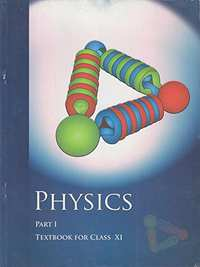Chapter 3: Motion in a Straight Line

Chapter 3: Motion in a Straight Line solutions [Pages 55 - 60]

Q 2 | Page 55

The position-time (x-t) graphs for two children A and B returning from their school O to their homes P and Q respectively are shown in Figure Choose the correct entries in the brackets below;

(a) (A/B) lives closer to the school than (B/A)

(b) (A/B) starts from the school earlier than (B/A)

(c) (A/B) walks faster than (B/A)

(d) A and B reach home at the (same/different) time

(e) (A/B) overtakes (B/A) on the road (once/twice).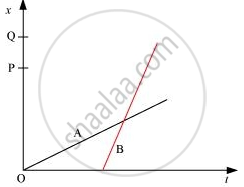Q 3 | Page 56

A woman starts from her home at 9.00 am, walks with a speed of 5 km h–1 on a straight road up to her office 2.5 km away, stays at the office up to 5.00 pm, and returns home by an auto with a speed of 25 km h–1. Choose suitable scales and plot the x-t graph of her motion.

Q 4 | Page 56

A drunkard walking in a narrow lane takes 5 steps forward and 3 steps backward, followed again by 5 steps forward and 3 steps backward, and so on. Each step is 1 m long and requires 1 s. Plot the x-tgraph of his motion. Determine graphically and otherwise how long the drunkard takes to fall in a pit 13 m away from the start.

Q 5 | Page 56

A jet airplane travelling at the speed of 500 km h–1 ejects its products of combustion at the speed of 1500 km h–1 relative to the jet plane. What is the speed of the latter with respect to an observer on ground?

Q 6 | Page 56

A car moving along a straight highway with a speed of 126 km h–1 is brought to a stop within a distance of 200 m. What is the retardation of the car (assumed uniform), and how long does it take for the car to stop

Q 7 | Page 56

Two trains A and B of length 400 m each are moving on two parallel tracks with a uniform speed of 72 km h–1 in the same direction, with A ahead of B. The driver of B decides to overtake A and accelerates by 1 m/s2. If after 50 s, the guard of B just brushes past the driver of A, what was the original distance between them?

Q 8 | Page 56

On a two-lane road, car A is travelling with a speed of 36 km h–1. Two cars B and C approach car A in opposite directions with a speed of 54 km h–1 each. At a certain instant, when the distance AB is equal to AC, both being 1 km, B decides to overtake A before C does. What minimum acceleration of car B is required to avoid an accident?

Q 9 | Page 56

Two towns A and B are connected by a regular bus service with a bus leaving in either direction every minutes. A man cycling with a speed of 20 km h–1 in the direction A to B notices that a bus goes past him every 18 min in the direction of his motion, and every 6 min in the opposite direction. What is the period of the bus service and with what speed (assumed constant) do the buses ply on the road?

Q 10 | Page 56

A player throws a ball upwards with an initial speed of 29.4 m s–1.

(a) What is the direction of acceleration during the upward motion of the ball?

(b) What are the velocity and acceleration of the ball at the highest point of its motion?

(c) Choose the = 0 m and = 0 s to be the location and time of the ball at its highest point, vertically downward direction to be the positive direction of x-axis, and give the signs of position, velocity and acceleration of the ball during its upward, and downward motion.

(d) To what height does the ball rise and after how long does the ball return to the player’s hands? (Take = 9.8 m s–2 and neglect air resistance).

Q 11.1 | Page 57

Read each statement below carefully and state with reasons and examples, if it is true or false;

A particle in one-dimensional motion with zero speed at an instant may have non-zero acceleration at that instant

Q 11.2 | Page 57

Read each statement below carefully and state with reasons and examples, if it is true or false;

A particle in one-dimensional motion with zero speed may have non-zero velocity,

Q 11.3 | Page 57

Read each statement below carefully and state with reasons and examples, if it is true or false;

A particle in one-dimensional motion with constant speed must have zero acceleration

Q 11.4 | Page 57

Read each statement below carefully and state with reasons and examples, if it is true or false;

A particle in one-dimensional motion with positive value of acceleration mustbe speeding up.

Q 12 | Page 57

A ball is dropped from a height of 90 m on a floor. At each collision with the floor, the ball loses one tenth of its speed. Plot the speed-time graph of its motion between = 0 to 12 s.

Q 13.1 | Page 57

Explain clearly, with examples, the distinction between magnitude of displacement (sometimes called distance) over an interval of time, and the total length of path covered by a particle over the same interval.

Q 13.2 | Page 57

Explain clearly, with examples, the distinction between magnitude of average velocity over an interval of time, and the average speed over the same interval. [Average speed of a particle over an interval of time is defined as the total path length divided by the time interval]. Show in both (a) and (b) that the second quantity is either greater than or equal to the first.

When is the equality sign true? [For simplicity, consider one-dimensional motion only].

Q 14 | Page 57

A man walks on a straight road from his home to a market 2.5 km away with a speed of 5 km h –1. Finding the market closed, he instantly turns and walks back home with a speed of 7.5 km h–1. What is the

(a) magnitude of average velocity, and

(b) average speed of the man over the interval of time (i) 0 to 30 min, (ii) 0 to 50 min, (iii) 0 to 40 min? [Note: You will appreciate from this exercise why it is better to define average speed as total path length divided by time, and not as magnitude of average velocity. You would not like to tell the tired man on his return home that his average speed was zero!]

Q 15 | Page 57

In Exercises 3.13 and 3.14, we have carefully distinguished between average speed and magnitude of average velocity. No such distinction is necessary when we consider instantaneous speed and magnitude of velocity. The instantaneous speed is always equal to the magnitude of instantaneous velocity. Why?

Q 16 | Page 57

Look at the graphs (a) to (d) Figure carefully and state, with reasons, which of these cannot possibly represent one-dimensional motion of a particle.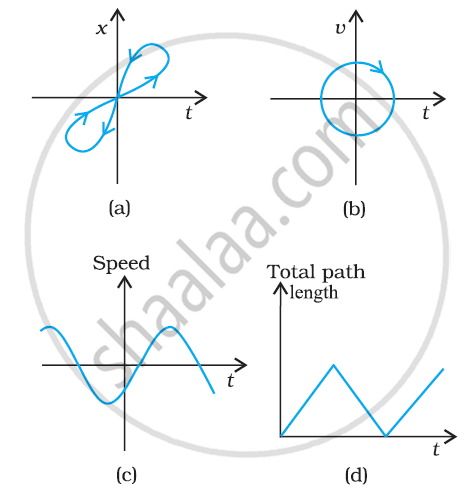Q 17 | Page 58

Figure shows the x-t plot of one-dimensional motion of a particle. Is it correct to say from the graph that the particle moves in a straight line for < 0 and on a parabolic path for > 0? If not, suggest a suitable physical context for this graph.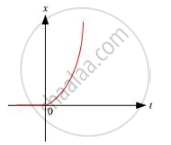Q 18 | Page 58

A police van moving on a highway with a speed of 30 km h–1 fires a bullet at a thief’s car speeding away in the same direction with a speed of 192 km h–1. If the muzzle speed of the bullet is 150 m s–1, with what speed does the bullet hit the thief’s car ? (Note: Obtain that speed which is relevant for damaging the thief’s car).

Q 19.1 | Page 58

Suggest a suitable physical situation for each of the following graphs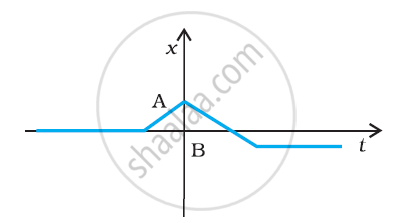Q 19.2 | Page 58

Suggest a suitable physical situation for each of the following graphs: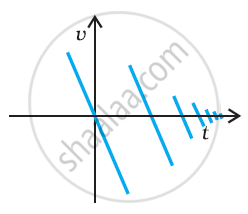Q 19.3 | Page 58

Suggest a suitable physical situation for each of the following graphs: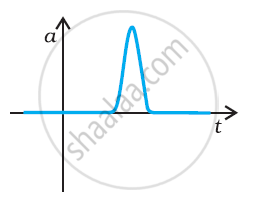Q 20 | Page 58

Figure gives the x-t plot of a particle executing one-dimensional simple harmonic motion. Give the signs of position, velocity and acceleration variables of the particle at = 0.3 s, 1.2 s, – 1.2 s.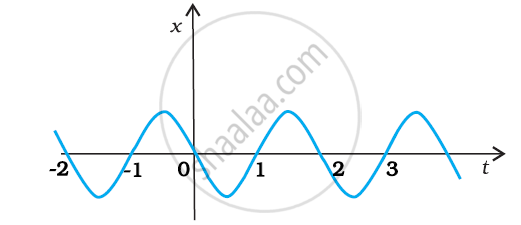Q 21 | Page 58

Figure gives the x-t plot of a particle in one-dimensional motion. Three different equal intervals of time are shown. In which interval is the average speed greatest, and in which is it the least? Give the sign of average velocity for each interval.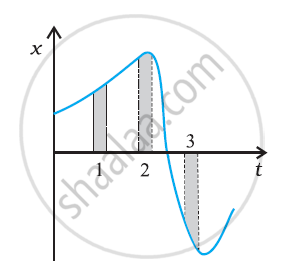Q 22 | Page 59

Figure gives a speed-time graph of a particle in motion along a constant direction. Three equal intervals of time are shown. In which interval is the average acceleration greatest in magnitude? In which interval is the average speed greatest? Choosing the positive direction as the constant direction of motion, give the signs of v and in the three intervals. What are the accelerations at the points A, B, C and D?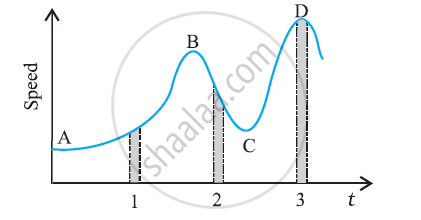Q 23 | Page 59

A three-wheeler starts from rest, accelerates uniformly with 1 m s–2 on a straight road for 10 s, and then moves with uniform velocity. Plot the distance covered by the vehicle during the nth second (n = 1,2,3….) versus n. What do you expect this plot to be during accelerated motion: a straight line or a parabola?

Q 24 | Page 59

A boy standing on a stationary lift (open from above) throws a ball upwards with the maximum initial speed he can, equal to 49 m/s. How much time does the ball take to return to his hands? If the lift starts moving up with a uniform speed of 5 m/s and the boy again throws the ball up with the maximum speed he can, how long does the ball take to return to his hands?

Q 25 | Page 59

On a long horizontally moving belt (Fig. 3.26), a child runs to and fro with a speed 9 km h–1 (with respect to the belt) between his father and mother located 50 m apart on the moving belt. The belt moves with a speed of 4 km h–1. For an observer on a stationary platform outside, what is the

(a) speed of the child running in the direction of motion of the belt ?.

(b) speed of the child running opposite to the direction of motion of the belt ?

(c) time taken by the child in (a) and (b) ?

Which of the answers alter if motion is viewed by one of the parents?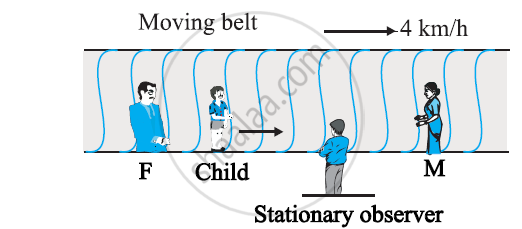Q 26 | Page 59

Two stones are thrown up simultaneously from the edge of a cliff 200 m high with initial speeds of 15 m/s and 30 m/s. Verify that the graph shown in Fig. 3.27 correctly represents the time variation of the relative position of the second stone with respect to the first. Neglect air resistance and assume that the stones do not rebound after hitting the ground. Take = 10 m/s2. Give the equations for the linear and curved parts of the plot.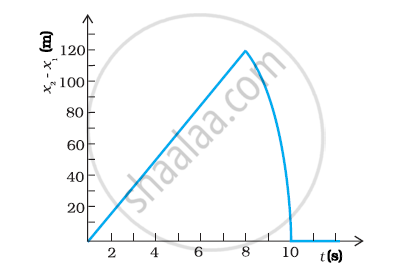Q 27 | Page 60

The speed-time graph of a particle moving along a fixed direction is shown in Fig. 3.28. Obtain the distance traversed by the particle between (a) = 0 s to 10 s, (b) = 2 s to 6 s.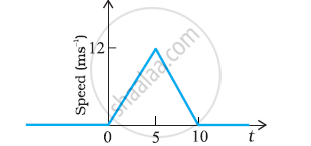What is the average speed of the particle over the intervals in (a) and (b)

Q 28 | Page 60

The velocity-time graph of a particle in one-dimensional motion is shown in Figure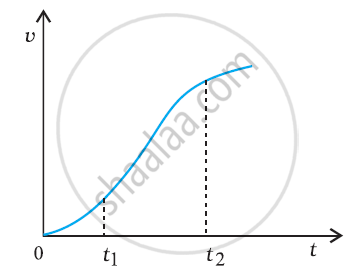Which of the following formulae are correct for describing the motion of the particle over the time-interval t2 to t1?

(a) x(t2) = x(t1) + v(t1)(t2–t1) + (1/2)a(t2–t1)2

(b) v(t2)= v(t1+ a(t2–t1)

(c) vAverage (x(t2– x(t1)) / (t2 – t1)

(d) aAverage (v(t2) – v(t1)) / (t2 – t1)

(e) x(t2= x(t1) + vAverage(t2 – t1) + (1/2)aAverage(t2 – t1)2

(f) x(t2) – x(t1) = area under the v–t curve bounded by the t-axis and the dotted line shown.

Chapter 3: Motion in a Straight Line Extra questions

Fill in the blanks by suitable conversion of units

1 m =..... ly

State the number of significant figures in the following:

2.64 × 1024 kg

In which of the following examples of motion, can the body be considered approximately a point object:

(a) a railway carriage moving without jerks between two stations.

(b) a monkey sitting on top of a man cycling smoothly on a circular track.

(c) a spinning cricket ball that turns sharply on hitting the ground.

(d) a tumbling beaker that has slipped off the edge of a table.

Chapter 3: Motion in a Straight Line

NCERT Physics Class 11NCERT solutions for Class 11 Physics chapter 3 - Motion in a Straight Line

NCERT solutions for Class 11 Physics chapter 3 (Motion in a Straight Line) include all questions with solution and detail explanation. This will clear students doubts about any question and improve application skills while preparing for board exams. The detailed, step-by-step solutions will help you understand the concepts better and clear your confusions, if any. Shaalaa.com has the CBSE Physics Textbook for Class 11 solutions in a manner that help students grasp basic concepts better and faster.

Further, we at Shaalaa.com are providing such solutions so that students can prepare for written exams. NCERT textbook solutions can be a core help for self-study and acts as a perfect self-help guidance for students.

Concepts covered in Class 11 Physics chapter 3 Motion in a Straight Line are Acceleration, Relative Velocity, Kinematic Equations for Uniformly Accelerated Motion, Elementary Concepts of Differentiation and Integration for Describing Motion, Uniform and Non-Uniform Motion, Position, Path Length and Displacement, Average Velocity and Average Speed, Instantaneous Velocity and Speed, Uniformly Accelerated Motion, Velocity - Time and Position-time Graphs, Motion in a Straight Line - Position-time Graph, Relations for Uniformly Accelerated Motion (Graphical Treatment).

Using NCERT Class 11 solutions Motion in a Straight Line exercise by students are an easy way to prepare for the exams, as they involve solutions arranged chapter-wise also page wise. The questions involved in NCERT Solutions are important questions that can be asked in the final exam. Maximum students of CBSE Class 11 prefer NCERT Textbook Solutions to score more in exam.

Get the free view of chapter 3 Motion in a Straight Line Class 11 extra questions for Physics and can use Shaalaa.com to keep it handy for your exam preparation

S